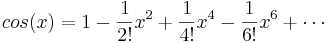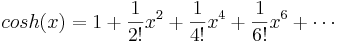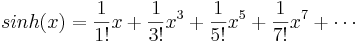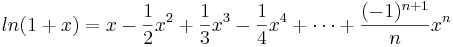# 7.4: Taylor Series

•• Contributed by Peter Woolf et al.
• Assistant Professor (Chemical Engineering) at University of Michigan

A Taylor Series is a representation of a function in the form of an infinite sum. Each term is calculated from using a derivative of the function as well as a factorial. The following equation is the defining equation of a Taylor series:

$f(x)=\int(a)-\frac{f^{\prime}(a)}{1 !}(x-a)+\frac{f^{\prime \prime \prime}(a)}{2 !}(x-a)^{2}+\frac{f^{(3)}(a)}{3 !}(x-a)^{3}+\cdots-\frac{f^{(n)}(a)}{n !}(x-a)^{n} \label{eq1}$

Where a is the point, around which the function is defined.

The Taylor approximation is most accurate at $$a$$, and becomes less precise as the distance from a increases. The following equation is the same as Equation \ref{eq1} mentioned above, in summation notation:

$f(x)=\sum_{n=0}^{\infty} \frac{f^{(n)}(a)}{n !}(x-a)^{n}$

We will first consider a simple example, the function, $$e^x$$, defined around the point, 0:

$e^{x}=e^{0}+\frac{1}{1 !}(x-0)+\frac{1}{2 !}(x-0)^{2}+\frac{1}{3 !}(x-0)^{3}+\cdots+\frac{1}{n !}(x-0)^{n}$

The numerators of each equation are all 1, because any level derivative of ex is ex, and ex, defined at 0 is always 1. The Taylor expansion simplifies to the following equation:

$e^{x}=1+\frac{x}{1 !}+\frac{x^{2}}{2 !}+\frac{x^{3}}{3 !}+\cdots+\frac{x^{n}}{n !}$

Also note that a Taylor series centered around 0 is called a Maclaurin series. In addition to the ex Maclaurin series, some other common, simplified Maclaurin series are listed here:These Maclaurin series can be modified to become Taylor series when the equation is not centered around zero.

Expansion of functions using Taylor series may be useful in derivations. Maclaurin series will be useful for when the function is being approximated for small values of x. Using an infinite number of terms is generally unnecessary to model the function around the center point. The first few terms of either a Taylor or Maclaurin series usually sufficiently approximate the function.

A stimulating discussion of Taylor series may be found in Comtet's "Calcul pratique des coefficients de Taylor d'une fonction algébrique" (Enseign. Math. 10, 267-270, 1964) as well as Whittaker and Watson's landmark treatise, "Forms of the Remainder in Taylor's Series." found in A Course in Modern Analysis, 4th ed.